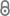# The complexity of equilibria for risk-modeling valuations

B. Monien, M. Mavronicolas, Theoretical Computer Science 634 (2016) 67–96.144-Monien_Mavronicolas-TCS2016_01.pdf 633.60 KB
Journal Article | English
Author
;
Abstract
Following the direction pioneered by Fiat and Papadimitriou in their 2010 paper , we study the complexity of deciding the existence of mixed equilibria for minimization games where players use valuations other than expectation to evaluate their costs. We consider risk-averse players seeking to minimize the sum V=E+R of expectationE and a risk valuationR of their costs; R is non-negative and vanishes exactly when the cost incurred to a player is constant over all choices of strategies by the other players. In a V-equilibrium, no player could unilaterally reduce her cost.Say that V has the Weak-Equilibrium-for-Expectation property if all strategies supported in a player's best-response mixed strategy incur the same conditional expectation of her cost. We introduce E-strict concavity and observe that every E-strictly concave valuation has the Weak-Equilibrium-for-Expectation property. We focus on a broad class of valuations shown to have the Weak-Equilibrium-for-Expectation property, which we exploit to prove two main complexity results, the first of their kind, for the two simplest cases of the problem:• Two strategies: Deciding the existence of a V-equilibrium is strongly NP-hard for the restricted class of player-specific scheduling games on two ordered links , when choosing R as (1)Var (variance), or (2)SD (standard deviation), or (3) a concave linear sum of even moments of small order.• Two players: Deciding the existence of a V-equilibrium is strongly NP-hard when choosing R as (1)γ⋅Var, or (2)γ⋅SD, where γ>0 is the risk-coefficient, or choosing V as (3) a convex combination of E+γ⋅Var and the concave ν-valuationν−1(E(ν(⋅))), where ν(x)=xr, with r≥2. This is a concrete consequence of a general strong NP-hardness result that only needs the Weak-Equilibrium-for-Expectation property and a few additional properties for V; its proof involves a reduction with a single parameter, which can be chosen efficiently so that each valuation satisfies the additional properties.
Publishing Year
Journal Title
Theoretical Computer Science
Volume
634
Page
67-96
LibreCat-ID

### Cite this

Monien B, Mavronicolas M. The complexity of equilibria for risk-modeling valuations. Theoretical Computer Science. 2016;634:67-96. doi:10.1016/j.tcs.2016.04.013
Monien, B., & Mavronicolas, M. (2016). The complexity of equilibria for risk-modeling valuations. Theoretical Computer Science, 634, 67–96. https://doi.org/10.1016/j.tcs.2016.04.013
@article{Monien_Mavronicolas_2016, title={The complexity of equilibria for risk-modeling valuations}, volume={634}, DOI={10.1016/j.tcs.2016.04.013}, journal={Theoretical Computer Science}, publisher={Elsevier}, author={Monien, Burkhard and Mavronicolas, Marios}, year={2016}, pages={67–96} }
Monien, Burkhard, and Marios Mavronicolas. “The Complexity of Equilibria for Risk-Modeling Valuations.” Theoretical Computer Science 634 (2016): 67–96. https://doi.org/10.1016/j.tcs.2016.04.013.
B. Monien and M. Mavronicolas, “The complexity of equilibria for risk-modeling valuations,” Theoretical Computer Science, vol. 634, pp. 67–96, 2016.
Monien, Burkhard, and Marios Mavronicolas. “The Complexity of Equilibria for Risk-Modeling Valuations.” Theoretical Computer Science, vol. 634, Elsevier, 2016, pp. 67–96, doi:10.1016/j.tcs.2016.04.013.
Main File(s)
File Name
144-Monien_Mavronicolas-TCS2016_01.pdf 633.60 KB
Access LevelClosed Access